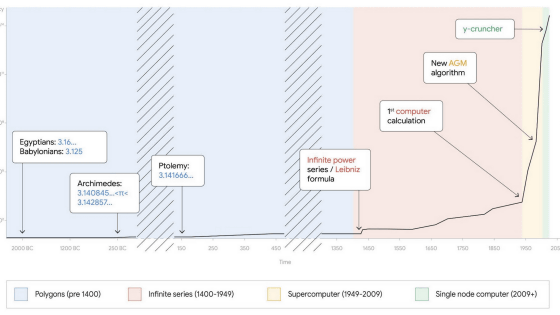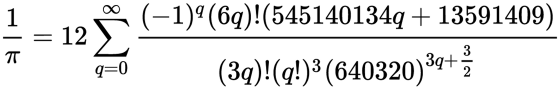# Finally, the 100 trillion digits of pi are revealed, and Google Cloud researchers spend about 5 months pursuing itPi , which indicates the ratio of the length of the circumference to the diameter of the circle, is a type of

irrational number that cannot be expressed as a fraction in which both the molecule and the denominator are integers. increase. The calculation to find the digit of such pi is inseparably related to the calculation of the computer, such as being used for the benchmark of the supercomputer. Emma Haruka Iwao , a developer and computer scientist at Google Cloud, announced on June 9, 2022 that she has finally calculated the pi to the 100th decimal place.

A bigger piece of the pi: Finding the 100-trillionth digit

Calculate 100 trillion digits of pi on Google Cloud | Google Cloud Blog

Calculations to find the digits of pi are done all over the world. The digit of pi was calculated to about 22.4 trillion digits in 2016, but on March 14, 2019, Mr. Iwao's team calculated it to 31.415.926.535897 digits. This number of digits is named after the circumference ratio '3.1415926535897 ……'.

It was announced on 'Pi Day' that it set a new record for pi, and it was achieved by a Google engineer from Japan --GIGAZINE.by Rob

Then, on January 29, 2020, computer scientist Timothy Marikan calculated 50 trillion digits, and on August 16, 2021, the Center for Data Analytics, Visualisation and Simulation at the University of Applied Sciences in Graubünden, Switzerland. (DAViS) announced that it took 108 days and 9 hours to calculate up to 62.8 trillion digits.

What are the specifications of a computer that set a new world record by calculating the circumference ratio to 62.8 trillion digits? --GIGAZINEThis is the second time that the Google Cloud research team has set a new world record for calculating pi digits. The fact that the calculated pi digits are increasing rapidly with the improvement of computers and algorithms can be seen in the graph below, which shows the number of pi digits (logarithmic display) on the vertical axis and the year on the horizontal axis. I understand well.According to Mr. Iwao, in this record update,

y-cruncher version 0.7.8 was adopted for the program, and Chudnovsky 's formula was adopted for the algorithm. The Chudnovsky formula is below and is known as a particularly efficient method for calculating pi.The compute node is n2-highmem-128 from Google Cloud. The OS is Debian 11, with 128 cores of virtual CPU and 864GB of memory, and it supports 100Gbps outward bandwidth. Also, of the 663TB of available storage, 515TB was used for calculation, the total amount of read data was 43.5P (peta) B, and the total amount of write data reached 38.5PB. The calculation started at 4:45:44 on October 14, 2021 in standard time, the calculation ended at 4:16:52 on March 21, 2022, and the calculation took 157 days 23 hours 31 minutes 7.651 seconds. I am.

The maximum capacity of a persistent disk that can be connected to a single virtual machine is 257TB, but since it was found that storage would use 554TB at the estimation stage, Mr. Iwao provided one compute node and a total of 64 iSCSI targets . It is said that it designed a cluster consisting of 32 storage nodes. Terraform was used to set up and manage the cluster, and a shell script was developed to perform tasks such as deleting old snapshots and resuming from snapshots, and automated backup acquisition every two days. Mr. Iwao has published a series of scripts on GitHub.

pi-delivery / pi-100t at main · GoogleCloudPlatform / pi-delivery · GitHub